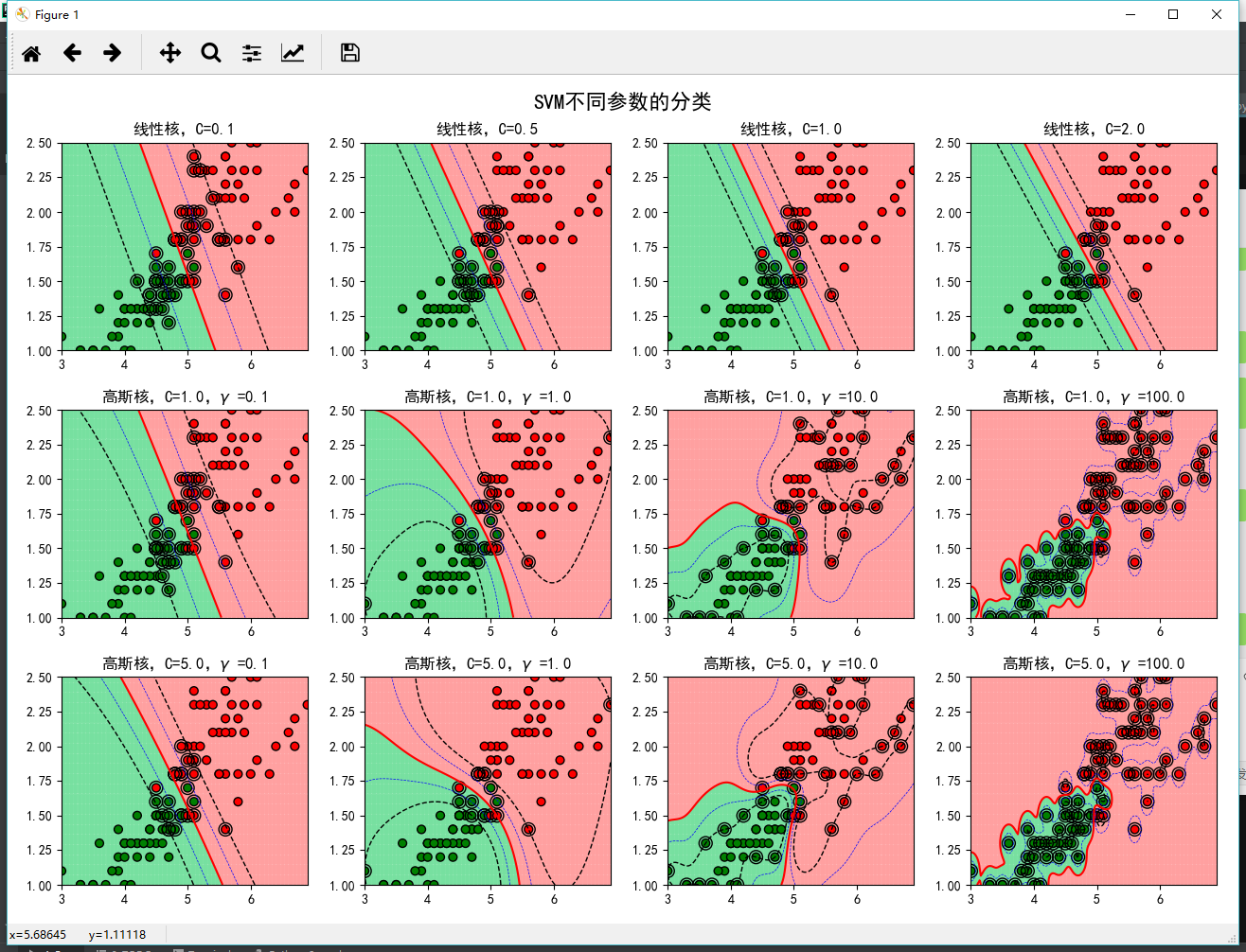## Scikit-learn 支持向量机库总结与简单实践

前两篇我们简单的探讨了SVM的原理，趁热我们来进行一些简单实践操作。磨刀不误砍柴工，先来认识下scikit-learn中集成的SVM算法库。scikit-learn中SVM的算法库分为两类，一类是分类的算法库，包括SVC， NuSVC，和LinearSVC 3个类。另一类是回归算法库，包括SVR， NuSVR，和LinearSVR 3个类。本篇我们先探讨下SVM分类算法库的使用。

1）scikit-learn SVM分类库概述
SVM分类算法库，包括SVC， NuSVC，和LinearSVC 3个类。SVC是基于libsvm实现，支持各种核函数，训练时间复杂度为 $O(m^2\times n)-O(m^3\times n)$，当样本量特别大时训练比较费时间；LinearSVC是基于liblinear实现，支持损失函数和正则的选择，只能处理线性可分问题，训练时间复杂度为 $O(m\times n)$，训练速度比SVC要快很多，特别在样本量特别大的时候；NuSVC和SVC非常相似，只是NuSVC可以通过超参数Nu控制支持向量的百分比，使用的场景非常少，我们就不做讨论了。

2）LinearSVC常用参数
LinearSVC类官方API：class sklearn.svm.LinearSVC(penalty=’l2’, loss=’squared_hinge’, dual=True, tol=0.0001, C=1.0, multi_class=’ovr’, fit_intercept=True, intercept_scaling=1, class_weight=None, verbose=0, random_state=None, max_iter=1000)[source]。下面介绍LinearSVC中我们经常会调整的一些参数：

• C，惩罚系数，默认为1
该参数就是我们在第一篇SVM中讲到的软间隔分类，用来权衡目标函数和松弛因子的超参数 $\lambda$。C值越大，支持向量的间隔越小，对错误的样本容忍度越小，泛化能力越小，因此当模型出现过拟合时，可以适当的降低C值。C值一般使用交叉验证方式进行选择。

• loss，损失函数参数，默认为‘squared_hinge’
LinearSVC独有，可以选择‘squared_hinge’或者 ‘hinge’。‘hinge’损失，即为第一篇SVM中讲到的hinge损失，‘squared_hinge’为hinge损失的平方。通常我们会使用‘hinge’。

• penalty，正则参数，默认 $l2$正则
LinearSVC独有，可以选择 $‘l1’$或者 $‘l2’$。如果我们需要产生稀疏化的系数，可以选 $L1$正则化。通常，保持默认设置。

• dual，是否对偶优化参数，默认为True
LinearSVC独有，优化目标函数是以原始形式还是采用拉格朗日对偶形式。当样本量比特征数多，此时采用对偶形式计算量较大，推荐dual设置为False。通常，将dual设置成False。

• class_weight， 样本权重参数，默认为None
当样本存在不平衡的情况下，需要设置该参数。 这里可以自己指定各个样本的权重，或者用‘balanced’，如果使用‘balanced’ ，则算法会自己计算权重，样本量少的类别所对应的样本权重会高。

• multi_class，多分类决策参数，默认’ovr’
LinearSVC独有，可以选择 ‘ovr’ 或者 ‘crammer_singer’ 。'ovr’的分类原则是将待分类中的某一类当作正类，其他全部归为负类，分别求得到每个类别作为正类时的概率，取概率最高的那个类别为最后的分类结果；‘crammer_singer’ 是优化所有类别的联合目标，准确性提升不明显，但计算量提升较高。通常，保持默认设置。

3）SVC常用参数
SVC类官方API：class sklearn.svm.SVC(C=1.0, kernel=’rbf’, degree=3, gamma=’auto_deprecated’, coef0=0.0, shrinking=True, probability=False, tol=0.001, cache_size=200, class_weight=None, verbose=False, max_iter=-1, decision_function_shape=’ovr’, random_state=None)[source]

• kernel，核函数参数，默认值为‘rbf’
该参数是为算法指定核函数的类型，SVC独有参数。可选的核函数有：
linear核函数 $K(x_i,x_j)=x_i\cdot x_j'$
poly核函数 $K(x_i,x_j)=(\gamma x_i\cdot x_j'+c)^d$
rbf核函数 $K(x_i,x_j)=exp(-\gamma ||x_i-x_j ||^2)$
Sigmoid核函数 $K(x_i,x_j)=tanh(\gamma x_i\cdot x_j +c)$
‘precomputed’选项，需要计算出所有的训练集和测试集的样本的核矩阵，K(x,z)直接在核矩阵中找对应的位置的值，矩阵大小为 $(n,n)$。核函数的选择，需要结合实际经验和数据情况。

• C，惩罚系数，默认为1
同LinearSVC参数C

• gamma，核函数参数，默认为‘auto_deprecated’
SVC独有参数，核函数为‘rbf’, ‘poly’ and ‘sigmoid’才需要调整该参数，分别对应核函数公式中的 $\gamma$。当取参数‘auto_deprecated’， $\gamma =\frac{1}{n_{features}}$ $\gamma$的作用和参数C类似，当模型出现过拟合时，减小γ值，当模型欠拟合，增大γ值。通常情况下，使用交叉验证的方式选择合适的 $\gamma$值。

• degree，核函数参数，默认为3
SVC独有参数，当核函数为’poly’时，该参数才有效，对应多项式核函数的阶数 $d$，默认为3阶多项式。阶数越高，模型越容易出现过拟合。通常情况下，使用交叉验证的方式选择合适的 $d$值。

• coef0，核函数参数，默认为0
SVC独有参数，当核函数为’poly’，'Sigmoid’时，该参数才有效，对应核函数中的截距项 $c$。通常情况下， $c$值保持默认设置。

• decision_function_shape，分类决策参数，默认为’ovr’
SVC独有参数，但和LinearSVC中的参数multi_class类似。可以选择 ‘ovo’, ‘ovr’,默认‘ovr’。
OvR(one ve rest)是指，无论多少分类，都看做二分类。即为，对于第K类的分类决策，把所有第K类的样本作为正例，其他所有样本都作为负例，然后在上面做二元分类，得到第K类的分类结果。其他类以此类推分别得到属于T个类别的概率，选择概率最高的作为最终的分类结果。
OvO(one-vs-one)则是每次在所有的T类样本里面选择两类样T1类和T2类出来，把T1和T2样本放在一起做二分类，选择概率最高的作为最终的分类结果，一共需要T(T-1)/2次分类。
因此，OvR相对简单，通常分类效果相对略差。而OvO分类相对精确，但分类速度没有OvR快。在做多分类任务时，一般将该参数设置为‘ovo’。

• class_weight， 样本权重参数，默认为None
同LinearSVC参数class_weight

• cache_size，缓存大小参数，默认200M
SVC独有参数，设置模型缓存大小参数。通常在样本量很大时，缓存大小会影响训练速度，如果机器内存大，可以设置512MB甚至1024MB。

• probability，是否输出概率，默认为‘False’
SVC独有参数，模型结果是否输出概率，使用的sigmoid函数计算样本概率。

4）SVM分类库经验总结

• 我们先试着使用线性核（LinearSVC比SVC(kernel=‘linear’)，快得多），特别是在特征很多或者数据集很大的情况。假如数据集不是很大，你也可以试着使用高斯RBF核，通常情况下表现都不错。如果你有空闲的时间和计算能力，你还可以使用交叉验证和网格搜索来试验其他的核函数，特别是有专门用于你的训练集数据结构的核函数。
• SVM算法对不同量纲的变量非常敏感，在进行模型训练之前，需要做标准化处理（使用Scikit-Learn的StandardScaler）。
• LinearSVC中，或者使用线性核的SVC，使用C=1或者较大的C，可能会导致模型无法收敛，陷入死循环。
• SVC不适合处理过大的数据。
• 核函数调参，使用grid_search和交叉验证选择最优超参数 $C,\lambda$

5）SVM算法库调参实践
为了更好的理解LinearSVC和SVC的超参数对模型的作用，我们下面结合一个具体的案例直观的感受超参数 $C,\lambda$对分类效果的影响，希望对大家在调整SVM算法库参数有一定的帮助。代码和数据已上传到我的Github

import numpy as np
import pandas as pd
from sklearn import svm
from sklearn.metrics import accuracy_score
import matplotlib as mpl
import matplotlib.colors
import matplotlib.pyplot as plt

if __name__ == "__main__":
x, y = data[[0, 1]], data

# 分类器
clf_param = (('linear', 0.1), ('linear', 0.5), ('linear', 1), ('linear', 2),
('rbf', 1, 0.1), ('rbf', 1, 1), ('rbf', 1, 10), ('rbf', 1, 100),
('rbf', 5, 0.1), ('rbf', 5, 1), ('rbf', 5, 10), ('rbf', 5, 100))

x1_min, x2_min = np.min(x, axis=0)
x1_max, x2_max = np.max(x, axis=0)
x1, x2 = np.mgrid[x1_min:x1_max:200j, x2_min:x2_max:200j]
grid_test = np.stack((x1.flat, x2.flat), axis=1)

cm_light = mpl.colors.ListedColormap(['#77E0A0', '#FFA0A0'])
cm_dark = mpl.colors.ListedColormap(['g', 'r'])
mpl.rcParams['font.sans-serif'] = ['SimHei']
mpl.rcParams['axes.unicode_minus'] = False
plt.figure(figsize=(13, 9), facecolor='w')
for i, param in enumerate(clf_param):
clf = svm.SVC(C=param, kernel=param)
if param == 'rbf':
clf.gamma = param
title = '高斯核，C=%.1f，$\gamma$ =%.1f' % (param, param)
else:
title = '线性核，C=%.2f' % param

clf.fit(x, y)
y_hat = clf.predict(x)
print('准确率：', accuracy_score(y, y_hat))

# 画图
print(title)
print('支撑向量的数目：', clf.n_support_)
print('支撑向量的系数：', clf.dual_coef_)
print('支撑向量：', clf.support_)
plt.subplot(3, 4, i+1)
grid_hat = clf.predict(grid_test)       # 预测分类值
grid_hat = grid_hat.reshape(x1.shape)  # 使之与输入的形状相同
plt.pcolormesh(x1, x2, grid_hat, cmap=cm_light, alpha=0.8)
plt.scatter(x, x, c=y, edgecolors='k', s=40, cmap=cm_dark)      # 样本的显示
plt.scatter(x.loc[clf.support_, 0], x.loc[clf.support_, 1], edgecolors='k', facecolors='none', s=100, marker='o')   # 支撑向量
z = clf.decision_function(grid_test)
print('clf.decision_function(x) = ', clf.decision_function(x))
print('clf.predict(x) = ', clf.predict(x))
z = z.reshape(x1.shape)
plt.contour(x1, x2, z, colors=list('kbrbk'), linestyles=['--', '--', '-', '--', '--'],
linewidths=[1, 0.5, 1.5, 0.5, 1], levels=[-1, -0.5, 0, 0.5, 1])
plt.xlim(x1_min, x1_max)
plt.ylim(x2_min, x2_max)
plt.title(title, fontsize=12)
plt.suptitle('SVM不同参数的分类', fontsize=16)
plt.tight_layout(1.4)
plt.show()（欢迎转载，转载请注明出处)